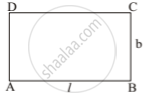# Sonam Has a Rectangular Garden. If the Length of the Garden is Reduced by 5 M and Breadth is Increased by 3 M, - Mathematics

MCQ

Sonam has a rectangular garden. If the length of the garden is reduced by 5 m and breadth is increased by 3 m, then the area of the garden gets reduced by 9 m2. However, if we increase the length by 3 m and breadth by 2 m, the area is increased by 67 m2. What is the length of the garden?

• 16 m

• 17 m

• 16.5 m

• 17.5 m

#### Solution

17 m

Explanation:Let ABCD is a rectangular garden with length

AB = l m and breadth BC = b m

Now, Area A = l . b.

(l - 5) × (b + 3) = lb - 9
3l - 5 b = 6  ....(i)

(l + 3) × (b + 2) = lb + 67
2l + 3b = 61    ...(ii)

From (i) and (ii), we get

9l + 10l = 18 + 305

19 l = 323

l = 323/19 = 17 "m"

Hence, length of the rectangle l = 17 m

Concept: Miscellaneous (Entrance Exam)
Is there an error in this question or solution?